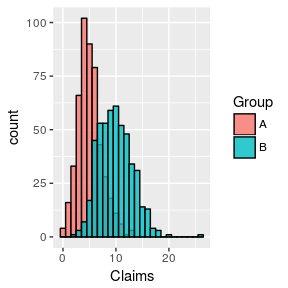# Function Helpers

#### 2017-06-05

Frequently, the imaginator package will need the user to pass a function as an argument to a simulation function. This function will then be called many times to simulate various quantities. For example, the ClaimsByLinkRatio function will generate random link ratios and so needs a function to do this. Actually, it needs more than one function so that each development lag gets its own random function.

To make this all a bit easier, imaginator includes some helper functions which create distribution functions and their parameters. For example, below we create two poisson functions, the second of which has twice the expected value of the first.

library(imaginator)
pois5 <- PoissonHelper(5)
pois10 <- PoissonHelper(10)
class(pois10)
##  "function"

These functions may now be used to generate random variables without having to specify the parameters of the distribution. The plot below shows a histogram of 500 simulations of each poisson function.

library(ggplot2)
set.seed(1234)

dfClaims <- rbind(data.frame(Group = "A", Claims = pois5(500))
, data.frame(Group = "B", Claims = pois10(500)))

plt <- ggplot(dfClaims, aes(Claims, fill = Group))
plt <- plt + geom_histogram(binwidth = 1, color = "black", alpha = 0.8, position = "identity")
plt